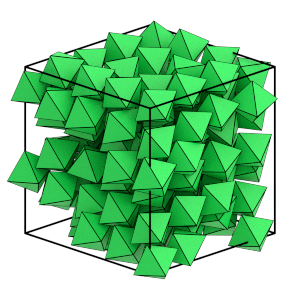# Equilibrating the System

## Overview

### Questions

• What is equilibration?

• How do I save simulation results?

### Objectives

• Explain the process of equilibration.

• Demonstrate using GSD to write the simulation trajectory to a file.

• Demonstrate best practices for move size tuning using Before and And Triggers.

## Boilerplate code

:

import math

import hoomd


The render function in the next (hidden) cell will render a snapshot using fresnel.

This is not intended as a full tutorial on fresnel - see the fresnel user documentation if you would like to learn more.

## Equilibration

So far, this tutorial has placed N non-overlapping octahedra randomly in a box and then compressed it to a moderate volume fraction. The resulting configuration of particles is valid, but strongly dependent on the path taken to create it. There are many more equilibrium configurations in the set of possible configurations that do not depend on the path. Equilibrating the system is the process of taking an artificially prepared state and running a simulation. During the simulation run, the system will relax to equilibrium. Initialize the Simulation first:

:

cpu = hoomd.device.CPU()
sim = hoomd.Simulation(device=cpu, seed=20)
mc = hoomd.hpmc.integrate.ConvexPolyhedron()
mc.shape['octahedron'] = dict(vertices=[
(-0.5, 0, 0),
(0.5, 0, 0),
(0, -0.5, 0),
(0, 0.5, 0),
(0, 0, -0.5),
(0, 0, 0.5),
])
sim.operations.integrator = mc


The previous section of this tutorial wrote the compressed system to compressed.gsd. Initialize the system state from this file:

:

sim.create_state_from_gsd(filename='compressed.gsd')


## Writing simulation trajectories

Save the system state to a file periodically so that you can observe the equilibration process. This tutorial previously used GSD files to store a single frame of the system state using either the GSD Python package or GSD.write. The GSD Writer (another operation) will create a GSD file with many frames in a trajectory.

We use the 'xb' mode to open the file to ensure that the file does not exist before opening it. If the file does exist an error will be raised rather than overwriting or appending to the file.

:

gsd_writer = hoomd.write.GSD(filename='trajectory.gsd',
trigger=hoomd.trigger.Periodic(1000),
mode='xb')
sim.operations.writers.append(gsd_writer)


## Tuning the trial move size

The previous section used the MoveSize tuner regularly during compression to adjust d and a to achieve a target acceptance ratio while the system density changed rapidly. Use it again during the equilibration run to ensure that HPMC is working optimally.

Move sizes should be tuned briefly at the beginning, then left constant for the duration of the run. Changing the move size throughout the simulation run violates detailed balance and can lead to incorrect results. Trigger the tuner every 100 steps but only for the first 5000 steps of the simulation by combining a Periodic and Before trigger with an And operation. Before returns True for all time steps t < value and the And trigger returns True when all of its child triggers also return True.

:

tune = hoomd.hpmc.tune.MoveSize.scale_solver(
moves=['a', 'd'],
target=0.2,
trigger=hoomd.trigger.And([
hoomd.trigger.Periodic(100),
hoomd.trigger.Before(sim.timestep + 5000)
]))
sim.operations.tuners.append(tune)

:

sim.run(5000)


Check the acceptance ratios over the next 100 steps to verify that the tuner achieved the target acceptance ratios:

:

sim.run(100)

:

rotate_moves = mc.rotate_moves
mc.rotate_moves / sum(mc.rotate_moves)

:

0.18988232534500957

:

translate_moves = mc.translate_moves
mc.translate_moves / sum(mc.translate_moves)

:

0.1901174817532493


## Equilibrating the system

To equilibrate the system, run the simulation. The length of the run needed is strongly dependent on the particular model, the system size, the density, and many other factors. Hard particle Monte Carlo self-assembly often takes tens of millions of time steps for systems with ~10,000 particles. This system is much smaller, so it takes fewer steps.

This cell may require several minutes to complete.

:

sim.run(1e5)


Here is the final state of the system after the run.

:

render(sim.state.get_snapshot())

:Is the final state an equilibrium state? The next section in this tutorial shows you how to analyze the trajectory and answer this question.